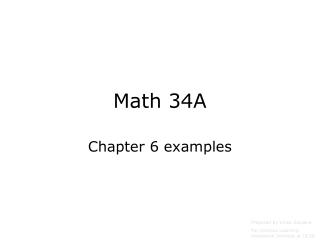Download PresentationMath 34A

# Math 34A - PowerPoint PPT PresentationDownload Presentation## Math 34A

- - - - - - - - - - - - - - - - - - - - - - - - - - - E N D - - - - - - - - - - - - - - - - - - - - - - - - - - -
##### Presentation Transcript

1. Math 34A Chapter 6 examples Prepared by Vince Zaccone For Campus Learning Assistance Services at UCSB

2. Example: In a meal plan at a college you pay a monthly membership fee, and then you receive all meals at a predetermined fixed price per meal. a) If 30 meals cost \$152.50 and 60 meals cost \$250, find the membership fee and the fixed price for a meal. b) Write a formula for the cost of a meal plan, C, in terms of the number of meals, m. c) Find the cost for 50 meals. d) Now find m in terms of C. e) Use part d) to determine the maximum number of meals you can buy on a budget of \$300.

3. Linear Functions can always be written as y = mx + b; m=slope; b=y-intercept

4. Linear Functions can always be written as y = mx + b; m=slope; b=y-intercept

5. “Proportional to” means “Equals a constant times” “Inversely Proportional to” means “Equals a constant divided by”